#### Nonconvex polygons and polyhedra

The method in Section 3.1.1 required nonconvex polygons to be represented as a union of convex polygons. Instead, a boundary representation of a nonconvex polygon may be directly encoded by listing vertices in a specific order; assume that counterclockwise order is used. Each polygon of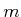vertices may be encoded by a list of the form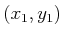,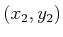,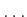,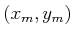. It is assumed that there is an edge between each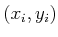and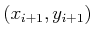for eachfromto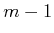, and also an edge betweenand. Ordinarily, the vertices should be chosen in a way that makes the polygon simple, meaning that no edges intersect. In this case, there is a well-defined interior of the polygon, which is to the left of every edge, if the vertices are listed in counterclockwise order.

What if a polygon has a hole in it? In this case, the boundary of the hole can be expressed as a polygon, but with its vertices appearing in the clockwise direction. To the left of each edge is the interior of the outer polygon, and to the right is the hole, as shown in Figure 3.5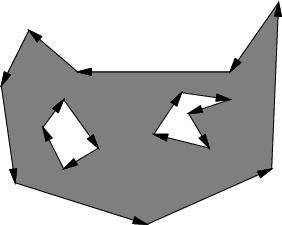Although the data structures are a little more complicated for three dimensions, boundary representations of nonconvex polyhedra may be expressed in a similar manner. In this case, instead of an edge list, one must specify faces, edges, and vertices, with pointers that indicate their incidence relations. Consistent orientations must also be chosen, and holes may be modeled once again by selecting opposite orientations.

Steven M LaValle 2020-08-14